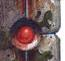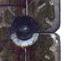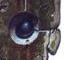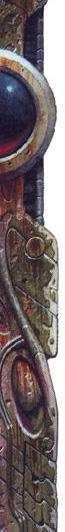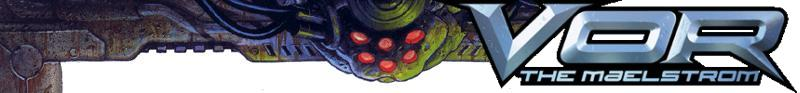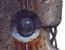Growlers Force PV Calculator

To avoid lots of repetition, the special abilities available to all growler bulls and chieftains are listed separately instead of as a list of options for each bull and chieftain. Abilities available to chieftains and/or specific breeds only are shown as options for those.

Total Force PV

Bluefangs

Bluefang Bull × 53 =
Awe-Inspiring Fury × +15 =
Belly Slam × +5 =
Excessive Blubber × +8 =
Smother Attack × +5 =
Tumble Attack × +10 =
Unrelenting Ferocity × +10 =
Bluefang Chieftain × 80 =
Awe-Inspiring Fury × +15 =
Belly Slam × +5 =
Earthshaker Roar × +15 =
Excessive Blubber × +8 =
Smother Attack × +5 =
Tumble Attack × +10 =
Unrelenting Ferocity × +10 =
What's That Smell? × +12 =
Bluefang Momma × 45 =
Foul Mood × +5 =
Vicious Claws × +8 =
Watchful Eye × +8 =
Bluefang Onehorn × 30 =
Extra Fat × +5 =
Powerful Strike × +8 =
Bluefang Pups × 6 =

Fireguts

Firegut Bull × 60 =
Awe-Inspiring Fury × +15 =
Extra Rocky Skin × +8 =
Fire Spray × +10 =
Magma Blast × +10 =
Scalding Steamcloud × +9 =
Unrelenting Ferocity × +10 =
Firegut Chieftain × 87 =
Awe-Inspiring Fury × +15 =
Earthshaker Roar × +15 =
Extra Rocky Skin × +8 =
Fire Spray × +10 =
Flaming Death × +20 =
Magma Blast × +10 =
Scalding Steamcloud × +9 =
Unrelenting Ferocity × +10 =
Firegut Momma × 45 =
Flame Breath × +8 =
Foul Mood × +10 =
Watchful Eye × +8 =
Firegut Onehorn × 30 =
Molten Blast × +10 =
Firegut Pups × 7 =

Razorbacks

Razorback Bull × 55 =
Awe-Inspiring Fury × +8 =
Frenzy × +12 =
Intimidating Presence × +4 =
Massive Horn × +8 =
Unrelenting Ferocity × +10 =
Razorback Chieftain × 85 =
Awe-Inspiring Fury × +8 =
Earthshaker Roar × +15 =
Frenzy × +12 =
Intimidating Presence × +4 =
Massive Horn × +8 =
Supertough Frame × +15 =
Unrelenting Ferocity × +10 =
Razorback Momma × 45 =
Foul Mood × +10 =
Vicious Claws × +8 =
Watchful Eye × +8 =
Razorback Onehorn × 30 =
Powerful Strike × +8 =
Steel Horn × +5 =
Razorback Pups × 7 =

Whitetusks

Whitetusk Bull × 55 =
Awe-Inspiring Fury × +15 =
Combat Lunge × +5 =
Horn-Tusk Smash × +8 =
Increased Knowledge × +3 =
Lethal Strike × +5 =
Unrelenting Ferocity × +5 =
Whitetusk Chieftain × 82 =
Awe-Inspiring Fury × +15 =
Combat Lunge × +5 =
Earthshaker Roar × +15 =
Horn-Tusk Smash × +8 =
Increased Knowledge × +3 =
Lethal Strike × +5 =
Unrelenting Ferocity × +5 =
Whitetusk Momma × 45 =
Foul Mood × +10 =
Vicious Claws × +8 =
Watchful Eye × +4 =
Whitetusk Onehorn × 30 =
Lunge Strike × +5 =
Lurkers × +8 =
Whitetusk Pups × 7 =

General Bull & Chieftain Abilities

Acid Belch × +9 =
Armored Plates × +15 =
Chomp Attack × +9 =
Concussive Bark × +5 =
Defensive Shockwave × +10 =
Defensive Tumbling × +8 =
Fearsome Roar × +2 =
Hammer Slam × +7 =
Hulking Brute × +15 =
Hurl Foe × +4 =
Maw Blast × +7 =
Mightly Leap × +12 =
Offensive Shockwave × +8 =
Quills × +4 =
Rend 'n' Throw × +10 =
Seasoned Fighter × +8 =

Special Characters

Blacktalon 125
Deathmaw 198
Goregut 185
Razorfang 212
Silvermane 140
Skulltusk 190
Total Force PV